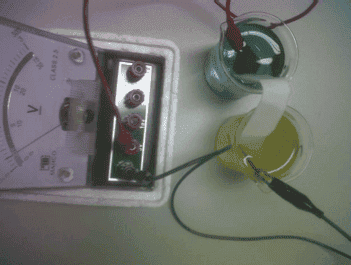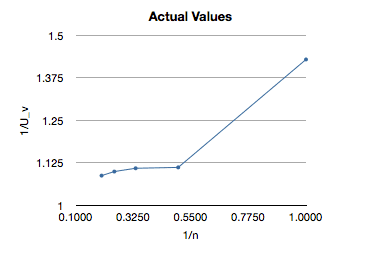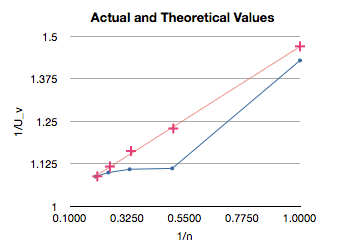# Salt Bridge & Voltaic Cells Experiment

I did an experiment testing the thickness of a salt bridge (made of filter paper soaked in potassium nitrate) on the voltage produced from Zn and Cu half cells.

But I have no idea how to explain my findings or whether the thickness even has an effect. Here are my results:

AVG Voltage
1 layer 7.1
2 layers 8.9
3 layers 9.0
4 layers 9.1
5 layers 9.2

Each strip of filter paper is 6 x 2 cm, stacked on top of each other to create the layers. According to the thread above, it shouldn't have an effect. But how do I attempt to explain the chemistry behind these results?

DrDu
What's the internal resistivity of your voltmeter?

I don't know what that means :(

But I'm using something like this. One's connected to the black and the other one's connected to the nearest red (I believe that's 0-3 V).

Last edited:
DrDu
Both your cell and your voltmeter have a resistance. The resistance of your cell depends on the number of layers (among other things). The reading of your voltmeter depends on both resistances.
I. e. if R1 is the resistance of your cell and R2 that of your voltmeter, and U is the true voltage of your cell then your voltmeter will only show U'=U R2/ (R1+R2).

DrDu
Doing a least squares fit to your data assuming that each strip has the same resistivity R1 I get:
U=10.1 V
R2/R1=2.4/0.9
7.3
8.5
8.9
9.2
9.4

DrDu
Btw. I don't quite understand how you can read an average voltage of 7.3-9.4 if your voltage scale ranges from 0 to 3 V (which is the range I would expect the voltage of a single Cu-Zn cell to fall in).

Borek
Mentor
Could be he reads a wrong scale.

Hi, sorry I haven't replied in a long, long while. Borek, yes I was reading the wrong scale :P

The real averages are:

1 layer filter paper 0.1400 mA
2 layer filter paper 0.1800 mA
3 layer filter paper 0.1804 mA
4 layer filter paper 0.1820 mA
5 layer filter paper 0.1840 mA

I understand that this may have something to do with the resistance, like what DrDu had mentioned. But I don't understand how he got the theoretical value from my results?

Both your cell and your voltmeter have a resistance. The resistance of your cell depends on the number of layers (among other things). The reading of your voltmeter depends on both resistances.
I. e. if R1 is the resistance of your cell and R2 that of your voltmeter, and U is the true voltage of your cell then your voltmeter will only show U'=U R2/ (R1+R2).

DrDu
I would explain to you in more detail, however, I still don't understand what you have done, exactly. I thought you were measuring voltage, at least you said so in your first post. However now you present some values in miliAmperes which is the unit of current, not of voltage.

This is my set-up:And I'm sorry, I read the wrong scale thing again. It's not mA, its in V, and the averages are:

0.700
0.900
0.902
0.910
0.920

DrDu
That makes more sense. First a question: Do you know the fabricate of the voltmeter? As I said before, it would be important to know some characteristics of the instrument.
Now to your question: There is a current I flowing in the circuit which is limited by the resistance R of the circuit and the voltage U of the cell according to Ohm's law I=U/R.
You want to determine U. The total resistance R is the sum of the resistance of the voltmeter R_V, the ionic solutions R_S and of the n salt bridges R_B. If the salt bridges are identical, the resistance of all the salt bridges R_B is 1/n times the resistance of a single bridge R_b. Hence R=R_V+R_S+R_b/n. I would guess that R_S is much smaller than the other two terms and can be neglected.

Now the voltage drop at the voltmeter U_v, i.e. the actual reading of your voltmeter is U_v=I R_V. Now you use I=U/R to obtain U=U_V R/R_V.
Hence if you plot 1/U_V over 1/n you should obtain a straight line whose value at x=1/n=0 is the voltage U of your cell (which is easy and what you should try out!).

Thank you so much DrDu!

I understood everything up to
Hence if you plot 1/U_V over 1/n you should obtain a straight line whose value at x=1/n=0 is the voltage U of your cell (which is easy and what you should try out!).

Also, how would I know what R_V and R_b is? Why did you arrange to find U, not U_v? Isn't U_v the value on a volt meter we want to find out?

DrDu
U_v, or even better U_v(n) are the readings of your voltmeter which you have and I thought you wanted to determine the actual voltage of the cell, U?
The slope of the line should be something like U R_b/R_v. U you have determined already and R_V you may know from the manual of the voltmeter, so you can determine the resistance of the salt bridge.

Ohh ok I get it now :)

So, if I expand the "R" and neglect the R_s, I would have:

U = [(R_v + R_b/n)U_v ] / Rv

But how would I know R_b? Or do I just use n = 1,2,3,4,5 and plot five lines with R_b on the x-axis?

DrDu
Almost,
write
1/U_V=1/U+R_b/(R_V U) 1/n.
so if you plot 1/U_V over 1/n, you get(hopefully) a straight line which cuts the y axis at 1/U and has a slope of R_b/(R_V U).

I'm sorry :( I still don't understand how we can use that to find U. What do I do with the graph?

DrDu
Plot 1 over the values from your post #10 over 1/1, 1/2, 1/3, 1/4, 1/4, 1/5, correspondingly. Try to lay a straight line through the points. Read off the value where it cuts the y axis. Then U should be the inverse of that value.

DrDu
Btw, don't you have access to a digital voltmeter ("multimeter") these tend to have a very high internal resistance. Would be interesting to test if the influence of the number of bridges disappears then.

Hi, I tried plotting my actual values on the 1/U_v and 1/n axis:I'm still not sure I understand your graph explanation. But if I know the gradient, then I can plot a line using the points (1/5, 1/0.92) and get something like this:So we can use this line to find the theoretical value (U_v) from looking at 1/U_v for each 1/n.

But I'd need to figure out the gradient, which is R_b/(R_v U). I would know U from y-intercept, and R_v from voltmeter (though the one I'd used is very overused, so I couldn't find the R_v anywhere on the box/manual: can we assume this is infinity? or at least a really large value).

Also, how do I figure out R_b?

In the graph above, the red line is actually the theoretical values you gave eariler:

Doing a least squares fit to your data assuming that each strip has the same resistivity R1 I get:
U=10.1 V
R2/R1=2.4/0.9
7.3
8.5
8.9
9.2
9.4

How did you figure this out? What assumptions did you make?

Thank you again for all this! :D

DrDu
Very good, I did that graph, too. The relation does not look too linear, what maybe is to be expected since the salt bridges are certainly not too homogeneous.
I was doing a least squares fit. E.g. in Excel you could chose to put a trend line when clicking on the graph. It can also show you the formula of the linear equation, the constant term is then the value 1/U you want.

Very good, I did that graph, too. The relation does not look too linear, what maybe is to be expected since the salt bridges are certainly not too homogeneous.
I was doing a least squares fit. E.g. in Excel you could chose to put a trend line when clicking on the graph. It can also show you the formula of the linear equation, the constant term is then the value 1/U you want.

Sorry, I'm just trying to write an explanation to how I came up with the theoretical value (the red line). So you just look at the trend lines from the values in post #10?

How do I use the stuff we did earlier with Ohm's law to explain this?